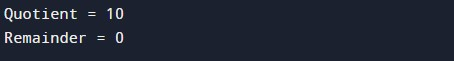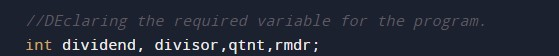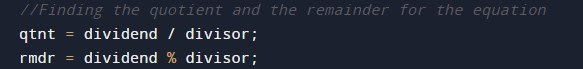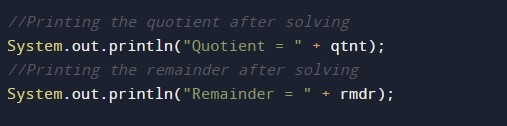# Java Program to Compute Quotient and Remainder

In this tutorial you will learn about the Java Program to Compute Quotient and Remainder and its application with practical example.

## Java Program to Compute Quotient and Remainder

In this tutorial, we will learn to create a Java program that will Compute the Quotient and the Remainder in Java programming.

## Prerequisites

Before starting with this tutorial, we assume that you are the best aware of the following Java programming topics:

• Operators in Java Programming.
• Basic Input and Output function in Java Programming.
• Basic Java Programming.

## What are Quotient and Remainder?

In the divide operation, the remaining part in the operation is a remainder. The answer part we get after the operation is a quotient.

## Program to Find Quotient and Remainder:-

In this program, we will first, declare the variables. Then we will assign the values to those variables. Now we will use the arithmetic expression to solve the problem. Finally at last, we will Print the answer.

With the help of this program, we can take input and Find Quotient and Remainder.

## Output:-In the above program, we have first initialized the required variable.• divisor = it will hold the input number value from the user.
• dividend= it will hold the input number value from the user.
• qtnt = it will hold the integer value of the Quotient.
• rmdr = it will hold the integer value of the Remainder.

Declaring the divisor and dividend from the user.Calculating the quotient and the remainder.Printing the output.In this tutorial we have learn about the Java Program to Compute Quotient and Remainder and its application with practical example. I hope you will like this tutorial.